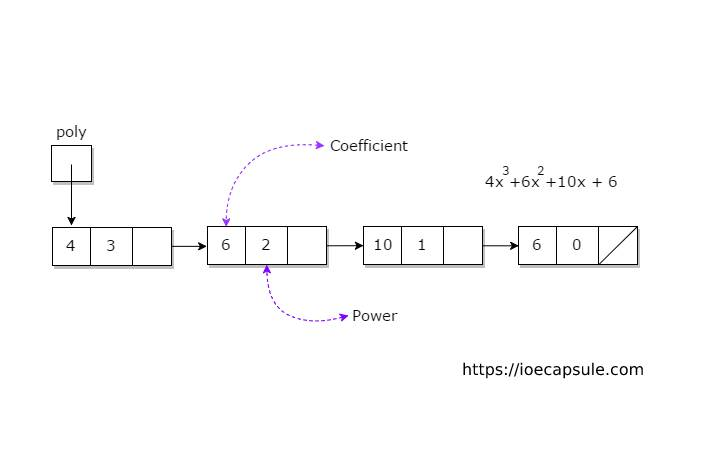Data Structures

Given two polynomials, represented by a linked list, write a function to add these polynomials and set the result to new polynomial represented by a linked list.

Adding polynomials means adding the coefficients of polynomial terms having equal power and appending the remaining terms of both polynomials.

``````
#include<iostream>
using namespace std;

class Node{
private:
int coeff;
int power;
char sign;
Node* next;
friend class Polynomial;
};

class Polynomial{
private:

void sort(){
bool sorted =  true;
while(sorted){
sorted = false;
while(inner->next != NULL){
if(inner->power < inner->next->power){
int tmpCoeff = inner->coeff;
int tmpPower = inner->power;
char tmpSign = inner->sign;

inner->coeff = inner->next->coeff;
inner->power = inner->next->power;
inner->sign = inner->next->sign;

inner->next->coeff = tmpCoeff;
inner->next->power = tmpPower;
inner->next->sign = tmpSign;

sorted = true;
}
inner = inner->next;
}
}
}
public:
Polynomial(){
}

void create(){

int coeff, power;
char again = 'n';
do{
cout<<"enter coefficient"<<endl;
cin>>coeff;
cout<<"enter power"<<endl;
cin>>power;

Node* node = new Node;
node->coeff = coeff;
node->power = power;
node->sign = coeff < 0 ? '-' : '+';
}else{
while(tmp->next != NULL){
tmp = tmp->next;
}
tmp->next = node;
}

sort();

do{
cout<<"enter another node ? y/n"<<endl;
cin>>again;
}while(again != 'n' && again != 'y');

}while(again != 'n');
}

Polynomial* result = new Polynomial;
bool compared = false;
while(a != NULL){
compared = false;
while(b != NULL){
if(b->power == a->power){
} else{
while(shifted->next != NULL){
shifted = shifted->next;
}
}
compared = true;
}
b = b->next;
}
if(!compared){
} else{
while(shifted->next != NULL){
shifted = shifted->next;
}
}
}
a = a->next;
}
//remaining from b
bool exists = false;
while(b != NULL){
while(a != NULL){
if(b->power == a->power){
exists = true;
}
a = a->next;
}
if(!exists){
} else{
while(shifted->next != NULL){
shifted = shifted->next;
}
}
}
b = b->next;
}
sort();
return *result;
}

void display(){
cout<<"Polynomial Not Set !"<<endl;
return;
}else{
char sign;
while(tmp != NULL){
cout<<tmp->coeff<<"x^"<<tmp->power<<" ";
}else{
cout<<tmp->sign<<abs(tmp->coeff)<<"x^"<<tmp->power<<" ";
}
tmp = tmp->next;
}
cout<<endl;
}
}

// ~Polynomial(){
// }

};

int main(){
Polynomial p1, p2;
bool exit = false;
do{
cout<<"===================================="<<endl;
cout<<"select option :"<<endl;
cout<<"1: set first polynomial"<<endl;
cout<<"2: set second polynomial"<<endl;
cout<<"3: display two polynomials"<<endl;
cout<<"5: for exit"<<endl;
int ch;
cin>>ch;
cout<<"===================================="<<endl;
switch(ch){
case 1:
p1.create();
break;
case 2:
p2.create();
break;
case 3:
p1.display();
p2.display();
break;
case 4:
break;
case 5:
exit = true;
cout<<"exiting..."<<endl;
break;
default:
cout<<"invalid selection !"<<endl;
break;
}
}while(!exit);
return 0;
}
``````

Feel free to modify the code as you like.